# 电性源瞬变电磁法在内蒙古多金属矿中的勘查实验Experiment of Electrical Source Transient Electromagnetic Method in Inner Mongolia Polymetallic Mineral Exploration

DOI: 10.12677/AG.2021.113030, PDF, HTML, XML, 下载: 29  浏览: 60  科研立项经费支持

Abstract: With the characteristics of convenient construction, large detection depth, and relatively high detection accuracy, the electrical source transient electromagnetic method is currently a research hotspot in new technologies and methods of geophysical prospecting. Because it has many advantages, so it has made great achievements in coal geology, but it is rarely used in the concealed ore bodies of polymetallic deposits. In this paper, based on the short-offset forward modeling program of the electric source and the definition of the global apparent resistivity, the short-offset transient electromagnetic test of a polymetallic mine in Inner Mongolia was carried out. The experimental results show that the receiving position of the tem is in the near field and the signal-to-noise ratio is high. At the same time, according to the effective skin depth formula of the electromagnetic field of the electric source, time-depth conversion is carried out to obtain sections of apparent resistivity of different buried depths. The treatment results show that the distribution of apparent resistivity of high and low resistivity is consistent with the curve of gravity anomaly. Combining of controlled source audio-frequency magnetotelluric inversion of apparent resistivity section, not only position of high and low resistance coincide well, and the electrical source transient electromagnetic can distinguish on the apparent resistivity section of small structure, lithologic interface, that is based on the electrical source short offset transient electromagnetic method the whole domain of the definition of apparent resistivity data interpretation, application in metal mine prospecting concealed ore body is feasible.

1. 引言

2. 瞬变电磁法原理

2.1. 瞬变电磁正演响应计算

${H}_{z}\left(\omega \right)=\frac{Idl}{2\pi }{\int }_{0}^{\infty }\frac{{\lambda }^{2}}{\lambda +{\mu }_{1}}{\text{e}}^{\lambda z}{J}_{1}\left(\lambda r\right)\frac{1}{{r}^{2}}\text{d}\lambda$ (1)

$\phi =\frac{2}{\pi }{\int }_{0}^{\infty }\mathrm{Im}\left[\frac{I}{2\pi }{\int }_{0}^{\infty }\frac{\lambda }{\lambda +{\mu }_{1}/{R}_{1}}{J}_{1}\left(\lambda r\right)\text{d}\lambda \right]\frac{\mathrm{cos}\left(\omega t\right)}{\omega }\text{d}t$ (2)

${H}_{z}\left(t\right)=\frac{1}{2\pi }{\int }_{-\infty }^{\infty }\frac{{H}_{z}\left(\omega \right)}{-i\omega }{\text{e}}^{i\omega t}\text{d}\omega$ (3)

2.2. 全域视电阻率定义

$dB=\phi \left(x,y,t,\rho ,{\gamma }_{0},{I}_{0}\right)$ (4)

$\begin{array}{c}\phi \left(\rho ,\text{const}\right)=\phi \left({\rho }_{0},\text{const}\right)+{\phi }^{\prime }\left({\rho }_{0},\text{const}\right)\left(\rho -{\rho }_{0}\right)+\frac{{\phi }^{″}\left({\rho }_{0},\text{const}\right)}{2!}{\left(\rho -{\rho }_{0}\right)}^{2}\\ \text{ }\text{\hspace{0.17em}}+\cdots +\frac{{\phi }^{\left(n\right)}\left({\rho }_{0},\text{const}\right)}{n!}{\left(\rho -{\rho }_{0}\right)}^{n}+{R}_{n}\left(\rho \right)\end{array}$ (5)

${\rho }_{1}=\frac{\phi \left(\rho ,\text{const}\right)-\phi \left({\rho }_{0},\text{const}\right)}{{\phi }^{\prime }\left({\rho }_{0},\text{const}\right)}+{\rho }_{0}$ (6)

${\rho }_{0}={\rho }_{1}$ 作为新的初值，在它的极小邻域内展开，并采取保留线性项的做法，我们又会得到新的 ${\rho }_{1}$，这样反复迭代下去，当迭代误差小于给定误差 $\epsilon$ 范围内，迭代终止：

$|\frac{dB-\phi \left({\rho }_{1},\text{const}\right)}{dB}|<\epsilon$ (7)

3. 地球物理特征Table 1. Statistical table of electrical parameters of mineral specimens

4. 电性源瞬变电磁试验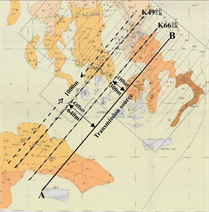Figure 1. Schematic diagram of electrical source TEM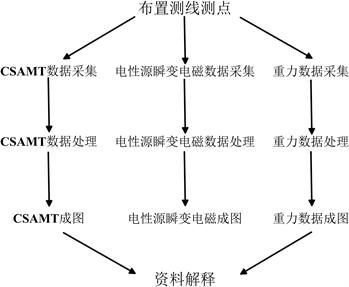Figure 2. Chart of flow

4.1. 探测结果及电性分布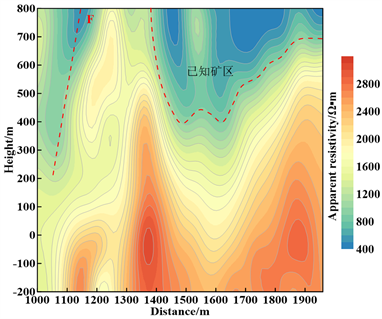Figure 3. Cross-sectional view of the global apparent resistivity of line K49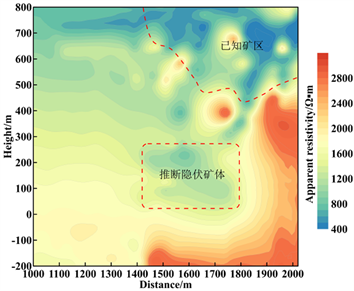Figure 4. Cross-sectional view of the global apparent resistivity of line K66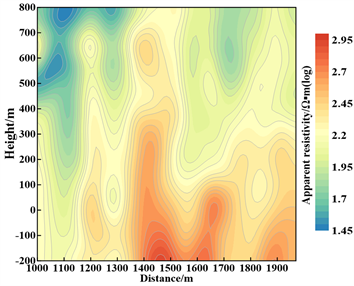Figure 5. CSAMT inversion of apparent resistivity profile on line K49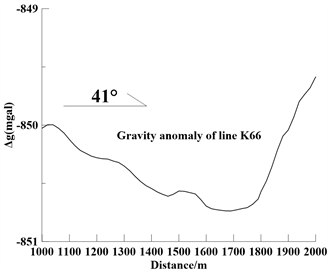Figure 6. Gravity Bouguer anomaly curve of line K66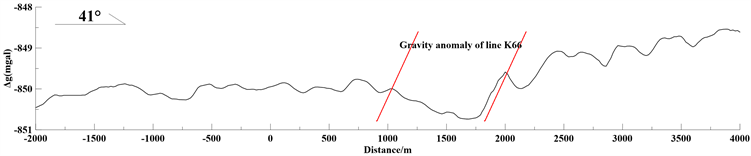Figure 7. Gravity Bouguer anomaly curve of K66 line

4.2. 多方法验证

K66线重力测量总长度为6 km，从总体曲线可以看到在1700 m位置出现重力异常负极值，如图7所示。为了便于对比图4所示的K66线全域视电阻率断面图，截取1000 m~2000 m进行单独成图。在图6所示1500 m位置，曲线向上凸起，形成突变，在图4位置对于高阻异常体向上凸起；在1500 m位置，重力异常曲线存在负极值，在图4全域视电阻率剖面上，显示低阻异常圈闭；在重力异常1800 m~2000 m位置，重力布格异常曲线上升，在图4全域视电阻率剖面上对应为高阻体向上延伸，因此，整体异常曲线更重力异常曲线吻合良好，说明全域视电阻率资料解释是可行，能很好的对地下岩性分布进行揭露，下一步结合钻孔进一步验证。

5. 结语

  方文藻, 李予国, 李貅. 瞬变电磁法探测原理[M]. 西安: 西北工业大学出版社, 1993: 311.  蒋邦远. 实用近区磁源瞬变电磁法勘探[M]. 北京: 地质出版社, 1998: 18.  李貅. 瞬变电磁测深的理论与应用[M]. 西安: 陕西科学技术出版社, 2002: 16.  底青云, 朱日祥, 薛国强, 等. 我国深地资源电磁探测新技术研究进展[J]. 地球物理学报, 2019, 62(6): 2128-2138.  牛之琏. 时间域电磁法原理[M]. 长沙: 中南大学出版社, 2007: 268-284.  薛国强, 于景邨. 瞬变电磁法在煤炭领域的研究与应用新进展[J]. 地球物理学进展, 2017, 32(1): 319-326.  何继善, 薛国强. 短偏移距电磁探测技术概述[J]. 地球物理学报, 2018, 61(1): 1-8.  薛国强, 陈卫营, 武欣, 等. 电性源短偏移距瞬变电磁研究进展[J]. 中国矿业大学学报, 2020(2): 1-12.  薛国强, 陈卫营, 周楠楠, 等. 接地源瞬变电磁短偏移深部探测技术[J]. 地球物理学报, 2013, 56(1): 255-262.  陈卫营, 薛国强, 崔江伟, 等. SOTEM响应特性分析与最佳观测区域研究[J]. 地球物理学报, 2016, 59(2): 739-748.  薛俊杰, 陈卫营, 王贺元. 电性源短偏移距瞬变电磁探测深度分析与应用[J]. 物探与化探, 2017, 41(2): 381-384.  薛国强, 闫述, 周楠楠. 偶极子假设引起的大回线源瞬变电磁响应偏差分析[J]. 地球物理学报, 2011, 54(9): 2389-2396.  李吉松, 朴化荣. 电偶源瞬变测深一维正演及视电阻率响应研究[J]. 物探化探计算技术, 1993, 15(2): 191-200.  白登海, Maxwell, A.-M., 卢健, 等. 时间域瞬变电磁法中心方式全程视电阻率的数值计算[J]. 地球物理学报, 2003, 46(5): 697-704.  陈卫营, 李海, 薛国强, 等. SOTEM数据一维OCCAM反演及其应用于三维模型的效果[J]. 地球物理学报, 2017, 60(9): 3667-3676.  卢云飞. 电性源短偏移距瞬变电磁数据一维反演方法研究[D]: [硕士学位论文]. 北京: 中国科学院大学, 2018: 18-39.  陈康. 电性源短偏移距瞬变电磁多源多分量探测方法研究[D]: [博士学位论文]. 北京: 中国科学院大学, 2018.  陈卫营, 薛国强, 崔江伟. 电性源瞬变电磁发射源形变对观测结果影响分析[J]. 地球物理学进展, 2015, 30(1): 126-132.  武军杰, 李貅, 智庆全, 等. 电性源地–井瞬变电磁异常场响应特征初步分析[J]. 物探与化探, 2017, 41(1): 129-135.  武军杰, 李貅, 智庆全, 等. 电性源地井瞬变电磁全域视电阻率定义[J]. 地球物理学报, 2017, 60(4): 1595-1605.  陈卫营, 薛国强. 接地导线源电磁场全域有效趋肤深度[J]. 地球物理学报, 2014, 57(7): 2314-2320.  智庆全. MTEM波场变换与偏移成像方法研究[D]: [硕士学位论文]. 西安: 长安大学, 2015.  张莹莹, 李貅, 姚伟华, 等. 多辐射场源地空瞬变电磁法多分量全域视电阻率定义[J]. 地球物理学报, 2015, 58(8): 2745-2758.  陈卫营, 薛国强. 接地导线源电磁场全域有效趋肤深度[J]. 地球物理学报, 2014, 57(7): 2314-2320.